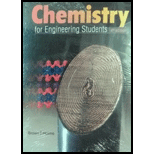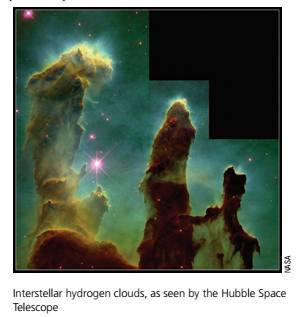Chapter 5, Problem 5.63PAEChemistry for Engineering Students

4th Edition
Lawrence S. Brown + 1 other
ISBN: 9781337398909Chemistry for Engineering Students

4th Edition
Lawrence S. Brown + 1 other
ISBN: 9781337398909
Textbook Problem

63 Clouds of hydrogen molecules have been detected deep in intersteller space, It is estimated that these clouds contain 1 × 10 10 hydrogen molecules per m3 and have a temperature of just 25 K. Using these data, find the approximate pressure in such a cloud.Interpretation Introduction

To determine:

Using the given data find the approximate pressure in such a cloud.

Explanation

No. of moles=No. of molecules6.022×1023 molecules

(Putting the value no of H2 molecules)

=1× 10 10 molecules6.022× 10 23 molecules=1.66×1014mole

V=1 m3T=25KR=8.314 Jmol1K1n=1

Still sussing out bartleby?

Check out a sample textbook solution.

See a sample solution

The Solution to Your Study Problems

Bartleby provides explanations to thousands of textbook problems written by our experts, many with advanced degrees!

Get Started

Find more solutions based on key concepts